Function Repository Resource:

# NInverseFourierSequenceTransform

Find a numerical approximation to the inverse Fourier sequence transform of a periodic function

Contributed by: Wolfram Research
 ResourceFunction["NInverseFourierSequenceTransform"][expr,ω,n] gives a numerical approximation to the inverse Fourier sequence transform of expr, evaluated at the integer n.

## Details and Options

Fourier sequence transform is also known as discrete-time Fourier transform (DTFT).
The numerical approximation to the inverse Fourier sequence transform of expr is by default defined to be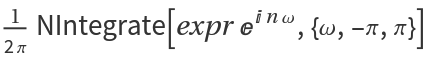.
Different choices for the definition of the inverse Fourier sequence transform can be specified using the option FourierParameters.
With the setting FourierParameters{a,b}, expr is assumed to have a period of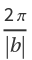, and the inverse Fourier sequence transform computed by ResourceFunction["NInverseFourierSequenceTransform"] is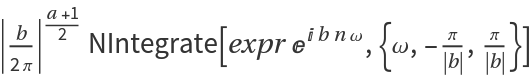.
The parameter b in the setting FourierParameters{a,b} must be numeric.
In addition to the option FourierParameters, ResourceFunction["NInverseFourierSequenceTransform"] can also accept the options available to NIntegrate. These options are passed directly to NIntegrate.

## Examples

### Basic Examples (2)

Numerical value for a sequence with a given Fourier sequence transform:

 In:=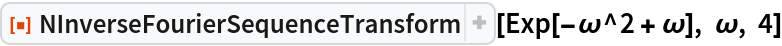Out=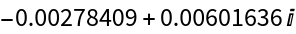Compare with the answer from symbolic evaluation:

 In:=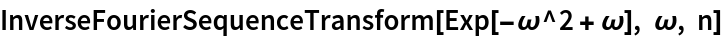Out=In:=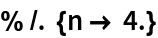Out=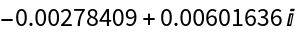## Requirements

Wolfram Language 11.3 (March 2018) or above

## Version History

• 1.0.0 – 12 April 2019